## Perform a One-Way Analysis of Variance (ANOVA) in Excel

The Analysis of Variance (ANOVA) has many varieties, but in essence, it has the purpose of evaluating whether factors are associated with any outcome values. And factors are categorical variables we use to group the outcome variables.

In this article, we will not be focusing on the underlying statistical principles and formulas. We will briefly touch on how we define the two hypotheses of ANOVA and will then show how to implement the model in Excel, as part of our financial analysis efforts.

## What is the ANOVA test?

ANalysis Of VAriance, written as ANOVA for short, is a statistical technique that compares sample populations based on their means and spread of the data. The model helps us answer the question of whether the means of two or more groups are significantly different.

We can rely on the Analysis of Variance (ANOVA) to evaluate the impact of different factors by comparing the means of different samples given by said factors.

ANOVA is a form of hypothesis testing, where we have the following two. The null hypothesis is that all sample means are equal or not significantly different in statistical terms.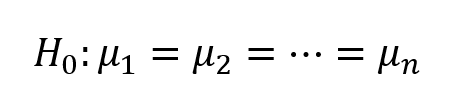And the alternative hypothesis is that at least one sample mean is significantly different from one of the others.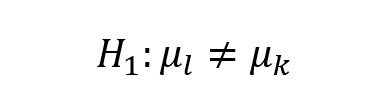Where we use l and k to denote numbers between 1 and n.

The biggest drawback of the ANOVA test is that it tells us whenever two groups have different sample means but does not help us identify which two groups exactly.

## Test Results Interpretation

When analyzing the results of the One-Way ANOVA test, we can use two measures to make our conclusions:

• Look at the p-value;
• Compare the F-test to the F-critical value.

A vital result of the model is the p-value, which only has meaning for the null hypothesis, stating all sample means are not significantly different (all groups have the same mean). In layman terms, we can interpret the p-value as the level of confidence we have that the null hypothesis is a plausible model for the data. Generally, if the p-value is below the significance level (denoted as Alpha), we will reject the null hypothesis.

If the ANOVA test shows an F-value that is larger than the F-critical value for the selected alpha level (normally 0.05 or 5%), we can reject the null hypothesis and accept the alternative (H1) – at least one sample mean is significantly different from one of the others.

## One-Way and Two-Way ANOVA

The main difference between One-Way and Two-Way ANOVA is the number of factors that we involve in our test. A One-Way (Single Factor) model helps us evaluate the equality between three or more sample means. On the other hand, a Two Factor ANOVA helps us assess the relationship and effect of two independent variables on the outcome (dependent variable).

## Perform Analysis of Variance in Excel

To better understand the concept, we will look at a practical example, where we can implement Analysis of Variance as part of our testing. An online retailer has three options to outsource deliveries of goods to its customers. The three companies have different pricing options for different locations and sizes of parcels. Therefore it’s hard to identify which one should be the primary choice whenever a new order is processed.

We have gathered data on the total shipping costs for the last ten orders processed with each of the three vendors.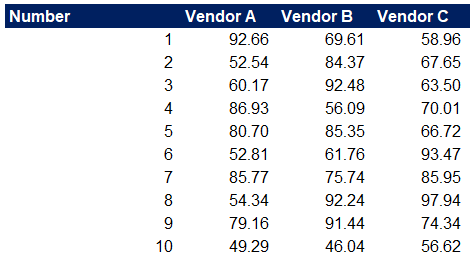We can start by calculating some descriptive statistics using Excel’s SUM, AVERAGE, and VAR.S functions.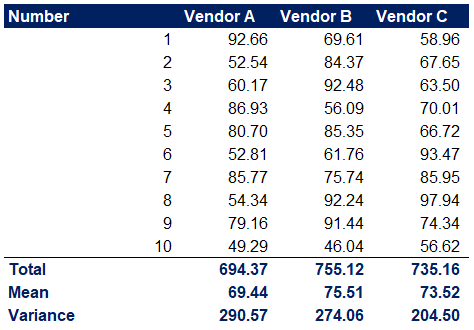Looking at the total cost for ten shipments and the average (mean) cost per order, we might be inclined to select Vendor A. It is a good idea to run more tests, as we are only looking at a sample of our processed orders. We want to be sure if the cost variations are a reason enough to prioritize Vendor A over the other two couriers.

We can run an ANOVA analysis to see if the three sample means are significantly different. To do so, we use the Data Analysis option in Excel, in the Data tab (If you don’t see it, you have to go into Excel Options -> Add-Ins and enable the Analysis ToolPak, which comes bundled with Excel). Once you run it, select the option for Anova: Single Factor and hit OK.This will open up a window to specify the required data for the ANOVA test.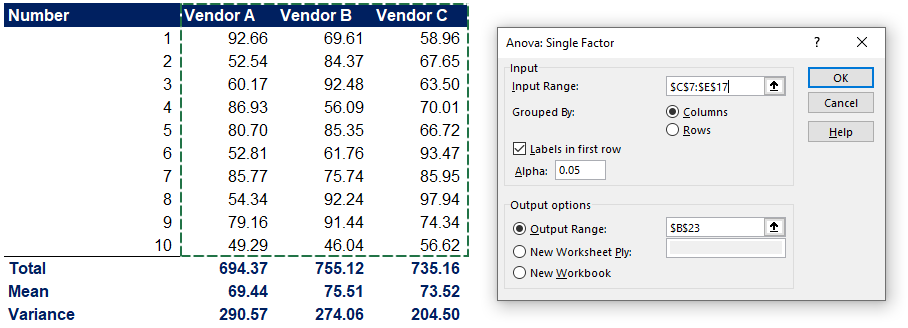Remember to specify whether Grouping is by Columns or Rows, check Labels in first row if you have selected the labels, and determine the significance level, Alpha. In most circumstances, I would go with 0.05 or 0.1, depending on the scenario. We can then run the model and look at the results. First, we get a summary of the descriptive statistics, which are the same as we already calculated above.And then we have the ANOVA test results.Following the approach we outlined above, we can form conclusions on whether the sample means (average cost per order) are significantly different between the three Vendors.

Let’s start with the p-value. The model shows a p-value of 0.69. This value suggests a high level of confidence that the null hypothesis gives an adequate model for the data.Next, let’s take a look at the F-test result and the F-critical value. The F value is 0.37, which is way below the critical F value of 3.35. These values also suggest that we have no reason to reject the null hypothesis.## Conclusion

In practice, the results we got suggest that the average cost per order is not significantly different between the three vendors. We must look at other factors to rate the suppliers. We can look at the shipping and handling time, customer feedback for the delivery service quality, and others.

The single factor ANOVA test is a fast and straightforward statistical method that can help us in our financial analysis endeavors. It can help us evaluate the equality, or the lack thereof, between various groups within our data. If we want to assess the relationship between two factors and how they affect the outcomes (dependent variable), we can also look at Two-Way ANOVA.## FCCA, FMVA

Hi! I am a finance professional with 10+ years of experience in audit, controlling, reporting, financial analysis and modeling. I am excited to delve deep into specifics of various industries, where I can identify the best solutions for clients I work with.

In my spare time, I am into skiing, hiking and running. I am also active on Instagram and YouTube, where I try different ways to express my creative side.